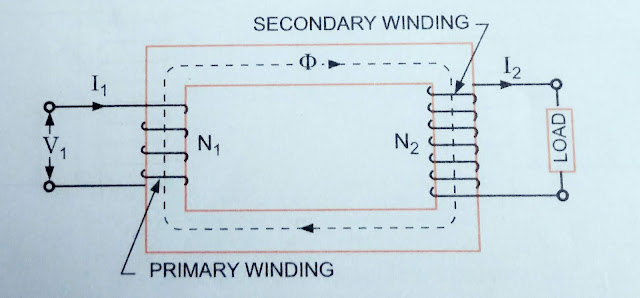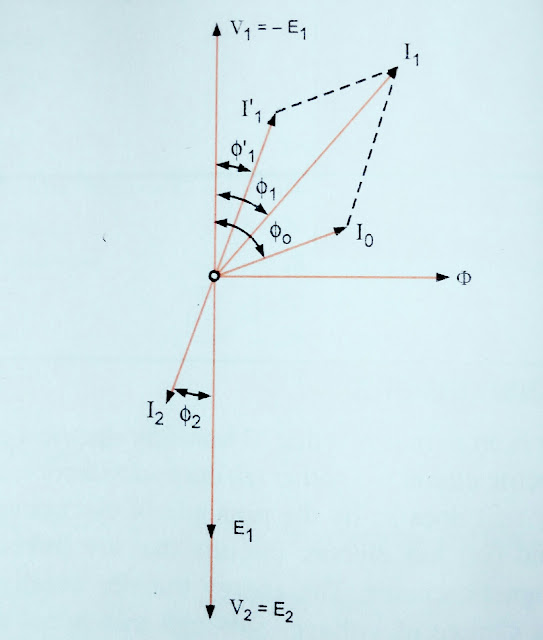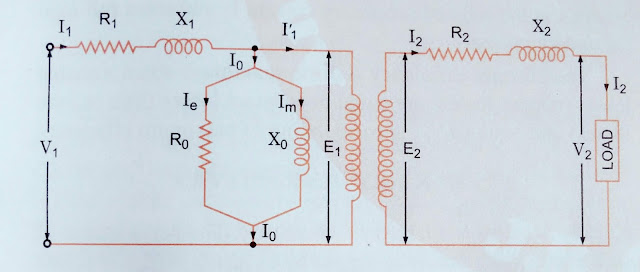### Ticker

6/recent/ticker-posts

# Transformer on load, Resistance and Leakage Reactance and more knowledge of Equivalent Circuit. [ CHAPTER :- 1 part :- B ]An Ideal Transformer ON load

The Primary Current,

I1 = I1 + I0 = KI2 + I0Phaser diagram For Inductive load

RESISTANCE AND LEAKAGE REACTANCE

In actual practice, both of the primary and secondary windings have got some ohmic resistance causing voltage drops and copper losses in the windings.
In actual practice, the total flux created does not Link both of the primary and secondary windings is but devided in to Three components namely the main of mutual fluxΦ Linking both of the primary and secondary winding, primary leakage flux ΦL1 linking with primary winding only and secondary leakage flux Φ L2 linking with secondary winding only. The primary leakage flux Φ L1 is Produced by Primary amps turns and is Proportional to primary Current, number of primary turns being fixed. The primary leakage flux Φ L1 is in phase with I1 produces and self induced EMF EL1 given as 2πfL1 I1 in the primary winding. The self induced EMF devided by primary current gives the reactance of primary and is denoted by X1.

EL1         2πfL1 I1
i.e.,   X1 = ------ = -------------- = 2πfL1
I1                 I1

Similarly leakage reactance of secondary

EL2         2πfL2 I2
X2 = ------- = --------------- = 2πfL2
E2                I2

A Transformer with magnetic leakage and winding resistance is equivalent to an ideal Transformer ( having resistance and leakage reactance ) having inductive and resistive coils connected in series with each winding as shown in fig.

When a practical Transformer is loaded

Induced EMF is Secondary winding
E2 = V2 + I2 ( R2 + jX2 ) = V2 + I2 Z2

Induced EMF in primary
E1 = E2 / k

And applied voltage to primary winding
V1 = -E1 + I1 ( R1 + jX1 ) = -E1 + I1 Z1

EQUIVALENT RESISTANCE AND REACTANCE

The Equivalent resistance and Reactances of Transformer referred to primary and secondary sides are given as follows;

Referred to primary Side
R2
Equivalent Resistance R01 = R1 + -------
K^2

X2
Equivalent Reactances X01 = X1 + -------
K^2

Referred to Secondary side

Equivalent Resistance R02 = K^2 R1 + R2
Equivalent Reactance X02 = K^2 X1 + X2
Where K is Transformation ratio.

Approximate Voltage drop in the transformer referred to Secondary

= I2 ( R02 CosΦ +- X02 sinΦ )

+ Ve sign for lagging power factor and -Ve sign leading power factor.

EQUIVALENT CIRCUIT

The Equivalent Circuit of any device can be quite helpful is predetermination of the behavior of the device under various condition of operation and it can be drawn if the equations describing it's behavior are known.EQUIVALENT CIRCUIT OF A TRANSFORMERS

Equivalent Circuit of a Transformer

Equivalent Circuit of a transformer having Transformation ratio
E2
K = ------- is shown in fig.
E1
Transformer Related more knowledge